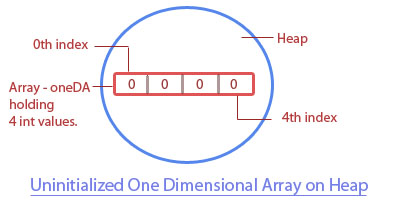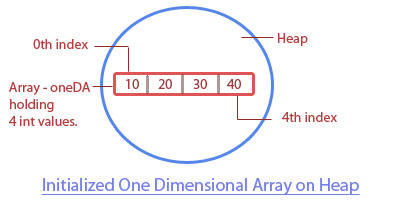< Prev
Next >

# Array in Java

Like almost all programming languages, Java language also provides us a capability by which we could hold a set of similar data types together, through its feature known as an array. An array allows us to hold multiple values of the same type.

These multiple values held by an array could be -
• primitive values of the same type , or
• object references of the same type.

We will explain how to create arrays holding these two different types of values in the upcoming section. But before that you should know that once an array is created, its size to hold multiple values cannot be modified, hence an array is static in nature.

## Types of array -

• One-dimensional array(1-D) is a single array that holds multiple values of the same type, i.e. the same type of primitive values or object references.

• Two-dimensional array(2-D) is an array containing multiple arrays within it, where all of these multiple arrays are holding values of the same type i.e. the same type of primitive values or the same type of object references.

## Note:

An Array is an object which is stored on the Heap memory.

We will understand about the one-dimensional array(1-D) in three separate parts -
• Array declaration
• Array construction
• Array initialization
Let's understand the steps involved in the declaration, construction, and initialization of a one-dimensional(1-D) array.

## Declaration of a 1-D array

We are going to declare a 1-D array that is going to hold primitive int values.

``````class A
{
public static void main(String... ar)
{
int[] array; //declaration of a 1-D array
}
}
``````

We have declared a one-dimensional array which doesn't exist on the heap yet.

## Construction of a 1-D array

An array object is constructed on the heap when its size is mentioned when it is created using the new keyword.

``````class A
{
public static void main(String... ar)
{
int[] array= new int; //Array object is constructed on the heap with size to hold 4 int values.
}
}
```
```In the example, the int array is constructed but its values aren't initialized. While the array is constructed on the heap to hold 4 int values but as we haven't initialized this array with those 4 values yet, hence, by default, this array has initialized itself with 4 zero values in it.

Note: If we don't initialize an array that holds at the time of its construction, it is initialized with a default value, depending on the array type. Please look at the table to know more -

Array type Default value
char '\u0000'
byte 0
int 0
float 0.0
double 0.0
Object null

## Initialization of a 1-D array

Array elements are stored using their index position in an array, with the first element stored at index zero and so on.
``````class A
{
public static void main(String... ar)
{
int[] oneDA= new int; //Array object is constructed on the heap when its size is mentioned at its creation.

oneDA=10;	//storing 10 at index 0 in array
oneDA=20;	//storing 20 at index 1 in array
oneDA=30;	//storing 30 at index 2 in array
oneDA=40;	//storing 40 at index 3 in array
}
}
```
```## Reading of a 1-D array using for loop

We can read the elements of an array using their index position in the array. For example -to read an element at an index 2 in an array named arr is as simple as -

``````System.out.println(arr);	//Reading 2nd index of this array gives us a value, 30
``````

But imagine when we have to read an array of 1000 elements, does it mean that we have to type in 1000 lines of code to read each element from its index? No, in order to easily read through all the elements of an array(irrespective of its size), we may use its public variable, length with the for-loop. Length variable tells us the total number of elements an array can hold, which is one more than the last index position at which an element is stored in an array.

For example- As you know that in an array, elements are stored starting at an index zero, hence if the length of an array is 5, the first element is stored at an index position 0 and the last element is stored at an index position 4.

``````class A
{
public static void main(String... ar)
{
char[] array= new char; //Array object is constructed on the heap when its size is mentioned at its creation.

array='a';
array='b';;
array='c';
array='d';

for(int i=0; i<array.length; i++) //accessing array elements using length variable in for-loop
{
System.out.println(array[i]);
}
}
}```
```

## Output -

``````a
b
c
d``````

## 1-D Array holding object references

So far, we read about one-dimensional array holding primitive values but an array can also be used to store object references (some people may call storing objects but in reality, only references of objects are stored).``````class A
{
public static void main(String... ar)
{
A[] array= new A; //Array holding 4 object references of type, A.

A ob1= new A();
A ob2= new A();
A ob3= new A();
A ob4= new A();

array = ob1;
array = ob2;
array = ob3;
array = ob4;

System.out.println("Length of an array "+ array.length); // length of an array.

for(int i=0; i<array.length; i++) //accessing array elements using length variable in for-loop
{
System.out.println(array[i]);
}
}
}
``````

## Output-

``````
Length of an array 4
A@70dea4e
A@5c647e05
A@33909752
A@55f96302``````